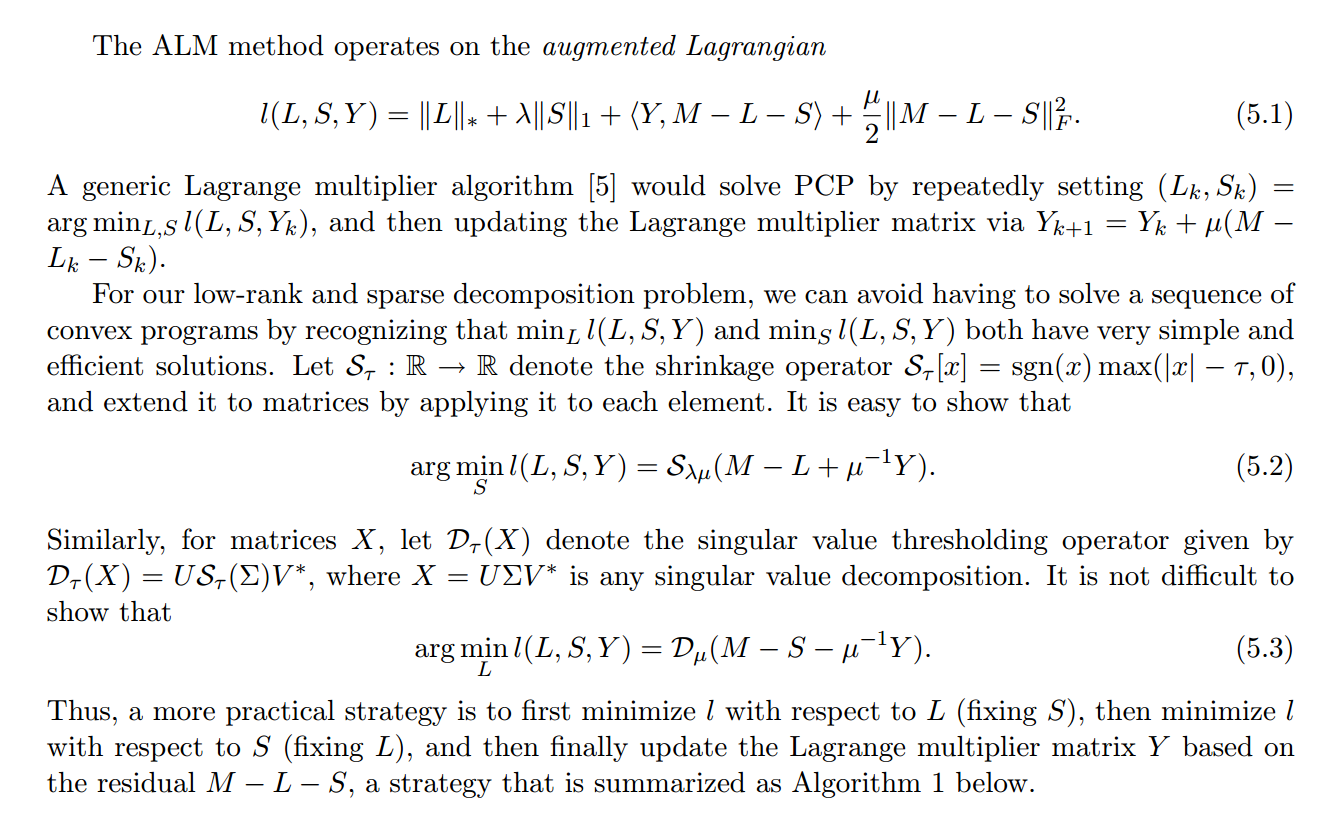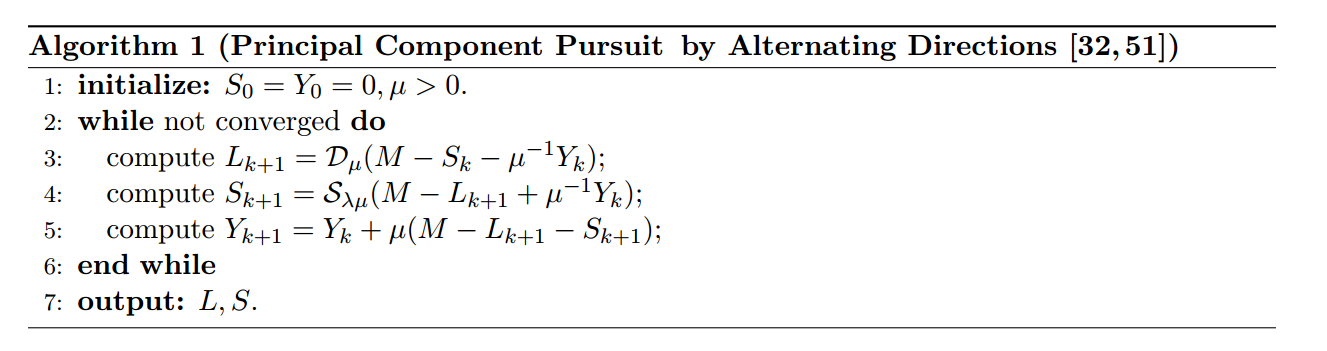# 从PCA到Robust PCA

Author: Steven Date: May 12, 2020 Updated On: May 19, 2020
Categories: 度量和表征
Tags: PCA RPCA
3.5k words in total, 13 minutes required.

### 回顾PCA

PCA即Principal components analysis，中文译作主成分分析或主元分析。通俗来讲，它是一种数据在度量空间上的降维方法：也就是说，我们在将数据进行表征空间的变换之后，能够最大化地用几个正交基来表示原有的数据（当然，这一过程可能是有损的）。当我们能用更少的维度来表达原有更多维度的数据时，降维的目的也即达成了。注意，主成分分析的结果是得到新的特征，而不是简单地舍弃原来的特征列表中的一些特征。

• 找到变异最大的新维度，以最大程度地区分不同数据点
• 这一新维度应该可以让我们预测和重建原始维度，重建或投影误差应该最小化

#### 协方差矩阵和特征向量的关系

• 特征向量重建了坐标系，相当于是一个旋转矩阵
• 缩放矩阵的平方等于对角线为特征值的对角矩阵

• 实对称矩阵不同特征值对应的特征向量必然正交。
• 设特征向量$\lambda$重数为$r$，则必然存在$r$个线性无关的特征向量对应于$\lambda\lambda$，因此可以将这$r$个特征向量单位正交化。

$\mathbf{P}$是协方差矩阵的特征向量单位化后按行排列出的矩阵，其中每一行都是$\mathbf{C}$的一个特征向量。如果设$\mathbf{P}$按照$\Lambda$中特征值的从大到小，将特征向量从上到下排列，则用$\mathbf{P}$的前$k$行组成的矩阵乘以原始数据矩阵$\mathbf{X}$，就得到了我们需要的降维后的数据矩阵$\mathbf{Y}$。

#### 算法

• 将原始数据按列组成$n$行$m$列矩阵$\mathbf{X}$
• 将$\mathbf{X}$的每一行（代表一个属性字段）进行零均值化，即减去这一行的均值
• 求出协方差矩阵
• 求出协方差矩阵的特征值及对应的特征向量
• 将特征向量按对应特征值大小从上到下按行排列成矩阵，取前$k$行组成矩阵$\mathbf{P}$
• $\mathbf{Y}=\mathbf{P}\mathbf{X}$即为降维到$k$维后的数据

#### 引申

PCA本质上是将方差最大的方向作为主要特征，并且在各个正交方向上将数据离相关，也就是让它们在不同正交方向上没有相关性。

### RPCA的引入

#### 优化算法ALMALM算法描述ALM算法

• shrinkage operator $S_\tau[x]=sgn(x)max(|x|−\tau,0)$
取$S$中的奇异值然后减去$\tau$，如果值比$\tau$小，就round为0。反之，就将两者的差反号，使得奇异值更接近0。总的来说这个shrinkage操作就是使得奇异值更小从而使它们更加稀疏。以实现找到一个sparse的$S$的目标。

• singular value thresholder $D$
就是先对矩阵做SVD分解，然后通过$S$的shrinkage操作使得奇异值变小之后，再重构$D_\tau(X)=U S_\tau (\Sigma)V^∗D_\tau(X) = U S_\tau(\Sigma)V^∗$得到低秩矩阵$L$的近似。

• 第3、4步的意思大概是，因为我们的目标是要把原矩阵分解成一个低秩矩阵$L$加上一个稀疏矩阵$S$，所以这里我们要不断让低秩矩阵等于原矩阵减去稀疏矩阵，同时让稀疏矩阵尽可能等于$M-L$。是一个典型的交替更新的方法。

### 扩展阅读

1. 1.
2. 2.
3. 3.
4. 4.Candès, E. J., Li, X., Ma, Y., & Wright, J. (2011). Robust principal component analysis?. Journal of the ACM (JACM), 58(3), 1-37.